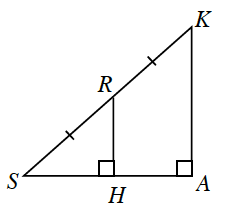### Home > CCG > Chapter Ch7 > Lesson 7.3.1 > Problem7-120

7-120.Examine the diagram at right.

1. Are the triangles in this diagram similar? Justify your answer using similarity transformations.

Draw $ΔSHR$ and $ΔSAK$ separately. Which angles are congruent?

2. What is the relationship between $HR$ and $AK$? Between $SH$ and $SA$? Between $SH$ and $HA$?

Compare $SR$ with $SK$. How are these lengths related? If the triangles are similar, then this relationship will be true for the other pairs of corresponding sides.

3. If $SK=20$ units and $RH=8$ units, what is $HA$?

Add the lengths to your diagram from part (a). If $SK=20$, what is $SR$?
Use the Pythagorean Theorem to calculate the unknown side length.